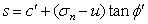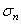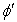# Mohr Coulomb

Shear strength of soil or rock is commonly modeled using the Mohr-Coulomb criterion, which is a linear failure criterion requiring two parameters - cohesion and friction angle.where:

s = shear strength

c’ = effective cohesion= total normal stress

u = pore pressure= effective angle of internal friction (phi)

The Mohr-Coulomb equation can be used for either total or effective stress conditions. For a total stress analysis, cohesion and friction angle are defined for total stress conditions. Pore water pressure is not considered, and the Mohr-Coulomb equation is simply:## Tensile Strength

See the Tensile Strength topic.